# ForceStrokeBehaviour

Force-stroke characteristic of the permeance model at constant current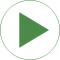# Information

This information is part of the Modelica Standard Library maintained by the Modelica Association.

Have a look at ConstantActuator and at PermeanceActuator for an explanation of both converter models.

Simulation of the force-stroke characteristic of the PermeanceActuator with a constant current I=3A and a forced armature movement (similar to measurements in reality) shows the dependency of both force-generating permeances G_ma and G_mb as well as inductance L on armature position x. Simulate for 8 s and plot vs. armature position feedX.flange_b.s (same physical quantities together in a common diagram for comparison):

```    feedX.flange_b.f            // force of permeance model (permeance of stator iron neglected in this model)
comparisonWithFEA.y      // force of FEA model with non-linear stator iron 1.0718
comparisonWithFEA.y      // force of FEA model with mu_rFe=const.=1000
actuator.g_ma.G_m           // permeance G_ma
actuator.g_mb.G_m           // permeance G_mb
actuator.L                  // inductance of permeance model
comparisonWithFEA.y      // inductance of FEA model for comparison (mu_rFe=const.=1000).
```

# Components (6)

ground actuator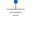Type: Ground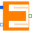Type: PermeanceActuator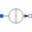Type: ConstantCurrent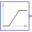Type: Ramp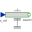Type: Position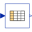Type: CombiTable1Ds Description: Column 1: position, col.2: force with non-linear stator iron, col.3: force with mu_rFe=const.=1000, col.4: inductance with mu_rFe=const.=1000